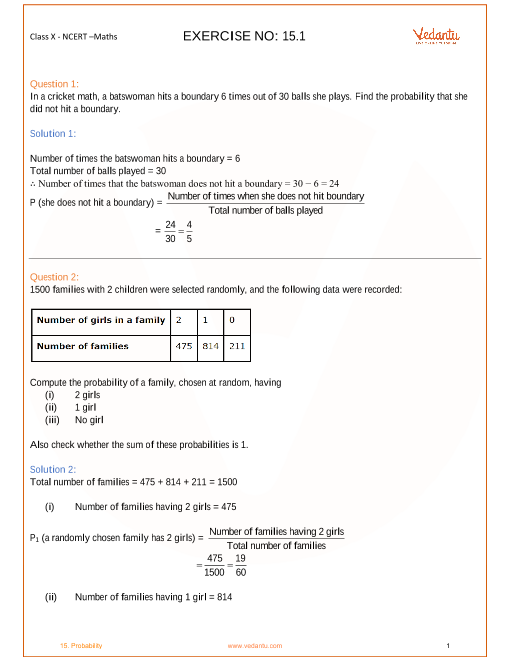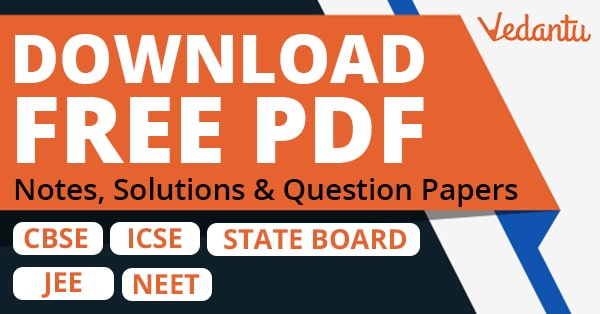NCERT Solutions for Class 10 Maths Chapter 15 Probability (Ex 15.2) Exercise 15.2

NCERT Solutions for Class 10 Maths Chapter 15 Probability (Ex 15.2) Exercise 15.2

Free PDF download of NCERT Solutions for Class 10 Maths Chapter 15 Exercise 15.2 (Ex 15.2) and all chapter exercises at one place prepared by expert teacher as per NCERT (CBSE) books guidelines. Class 10 Maths Chapter 15 Probability Exercise 15.2 Questions with Solutions to help you to revise complete Syllabus and Score More marks. Register and get all exercise solutions in your emails.